LemmaSS-cone.mw

 > restart;

 > with( plots ):

 > with( plottools ):

 >

Shrinking Sphere Problem

Derivation of General Formula for Intersection of S and S_r,

and Its Projection from the Top of S_r onto the z=0 Plane

When S is a Cone

9 February 2007

 >

Initial Configuration: the S (cone) and S_r (sphere) and the point P

 > S  := (a,b) -> x^2+(y-a)^2=(z-b)^2;  # fixed surface

 > Sr := r -> x^2+y^2+z^2=r^2;      # shrinking sphere

 > P  := r -> [ 0, 0, r ];          # top of shrinking sphere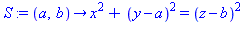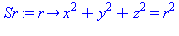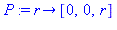>

 > plotP  := r     -> plot3d( P(r), x=-1..1, y=-1..1, style=point, symbol=circle, symbolsize=10, color=blue ):

 > plotS  := (a,b) -> implicitplot3d( S(a,b), x= -2*b..2*b, y=a-2*b..a+2*b, z=-b..b,                                   color=pink, style=patchnogrid, transparency=0.8, grid=[25,25,25] ):

 > plotSr := r     -> implicitplot3d( Sr(r), x=-r..r, y=-r..r, z=-r..r,                                   color=cyan, style=patchnogrid, transparency=0.8 ):

 > P1 := (r,a,b)   -> display( [plotP(r),plotS(a,b),plotSr(r)],                            axes=normal, labels=["x","y","z"], orientation=[25,65], args[4..-1] ):

 > P1(1,1,1, scaling=constrained);

 >

Construction of Q: Intersection of S and S_r

The intersection between these two spheres is a circle, parallel to the x=0 plane.

 > Intersection := [allvalues( solve( {S(a,b),Sr(r)}, {x,y,z} ) )] ;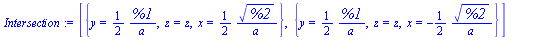There are two parts to this solution.

 > xPOS := p->evalb( eval(x, eval(p,[r=1.,a=1.,b=1.,z=0.]))>0 ):

 > Q := [ unapply( eval( [x,y,z], select( xPOS, Intersection )[] ), [z,r,a,b] ),

 > unapply( eval( [x,y,z], remove( xPOS, Intersection )[] ), [z,r,a,b] ) ]:

 > piecewise( x>0, Q(z,r,a,b), x<0, Q(z,r,a,b) );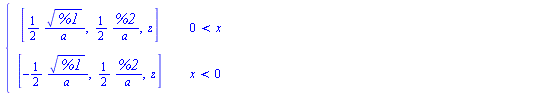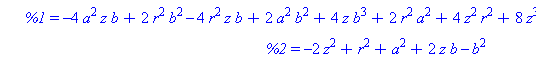>

To construct the projection from the top of the shrinking sphere through Q onto the z=0 plane, the parameterization of Q can be done in terms of z, ranging from the lowest value of 0 to the maximum value of

 > zM := (r,a,b) -> b*r/sqrt(a^2+b^2);(and back down to zero). Actually, the intersection exists for all z from -zM to zM (with x>0) and back down to -zM (with x<0)

 >

This curve is not easily identified.

 > plotQ := (r,a,b) -> display( [seq(

 > spacecurve( Q[i](z,r,a,b), z=-zM(r,a,b)..zM(r,a,b), color=gold, thickness=2 ),

 > i=1..2 )] ):

 > P2    := (r,a,b) -> display( [plotP(r),plotS(a,b),plotSr(r),plotQ(r,a,b)],                           axes=normal, labels=["x","y","z"], orientation=[45,60], scaling=constrained ):

 > P2(1,1,1);

 >

Construction of R: Projection of Q, from P, onto z=0 plane

For each angle theta, the lines passing through P and the point Q(theta) can be parameterized in terms of the (scaled) distance measured along this line.

 > LinePQ  := [seq( unapply( expand( (1-alpha)*P(r) + alpha*Q[i](z,r,a,b) ), [alpha,z,r,a,b] ), i=1..2 )]:

 > piecewise( x>0, LinePQ(alpha,z,r,a,b), x<0, LinePQ(alpha,z,r,a,b) );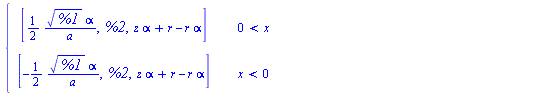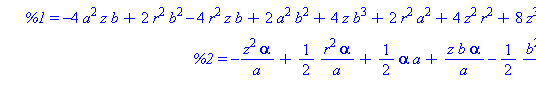>

The value of the parameter alpha when these lines hit the z=0 plane are given by

 > alpha0   := unapply( [simplify( solve( LinePQ(alpha,z,r,a,b)=0, alpha ) ) assuming a>0, r>0][],                     [z,r,a,b] );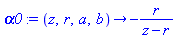>

Thus, the parametric representation of of the projected curve, R, in the z=0 plane is

 > R := [seq( unapply( [simplify( LinePQ[i](alpha0(z,r,a,b),z,r,a,b) ) assuming a>0, b>0, r>0][], [z,r,a,b] ), i=1..2 )]:

 > piecewise( x>0, R(z,r,a,b), x<0, R(z,r,a,b) );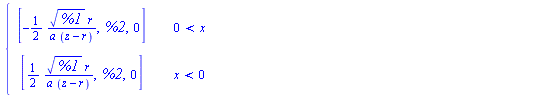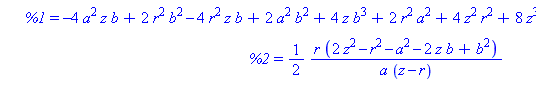>

This completes the constructions needed to put all of this together in one animation.

 > plotR := (r,a,b) -> display( [seq( spacecurve( R[i](z,r,a,b), z=-zM(r,a,b)..  zM(r,a,b), numpoints=201, color=red, thickness=1 ), i=1..2 )] ):

 > P3    := (r,a,b) -> display( [P2(r,a,b),plotR(r,a,b)] ):

 > animQ := (r,a,b) -> display( [seq( animate( spacecurve, [LinePQ[i](alpha,z,r,a,b), alpha=0..alpha0(z,r,a,b)], z=-zM(r,a,b)..  zM(r,a,b),                                      color=blue, thickness=2, orientation=[25,65], background=P3(r,a,b),                                      scaling=constrained, frames=21 ), i=1..2 )] ):

 > P3(1/2,1,1);

 >

 > animQ(1,1,1);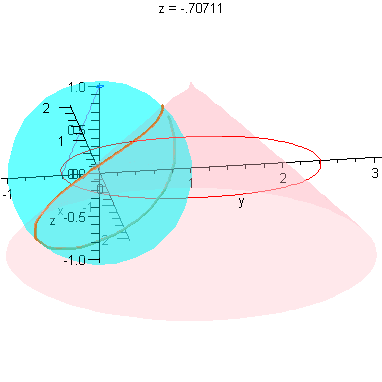> animQ(1/2,1,1);

 >

Limit as r -> 0

These plots already illustrate the rapid convergence of every point on the curves R - except the one on the x-axis - to the origin (as r->0). Let's look at the parametric form of R. The three components are (for x>0):

 > X,Y,Z := R(zeta,r,a,b)[]: x=X; y=Y; z=Z;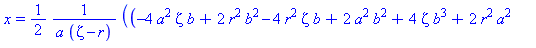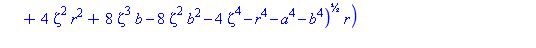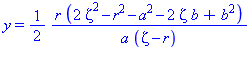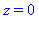>

Notice that, since a<>0, the maximum value of the parameter is strictly less than r. Hence, there are no indeterminate forms, and each of the above expressions converges to 0 (as r->0).

 > map( limit, [X,Y,Z], r=0, right );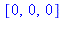>

We close with an animation that shows this convergence.

 > #to3d := transform( (x,y)->[x,y,0] ):

 > #plotR0 := a -> to3d( implicitplot( x^2 + (y-2*a)^2 = 4*a^2, x=-2*a..2*a, y=0..4*a, color=green ) ):

 > animR := (a,b) -> animate( P3, [1-r,a,b], r=0..1, frames=20, numpoints=201, paraminfo=false ):#, background=plotR0(a) ):

 > animR(1,1);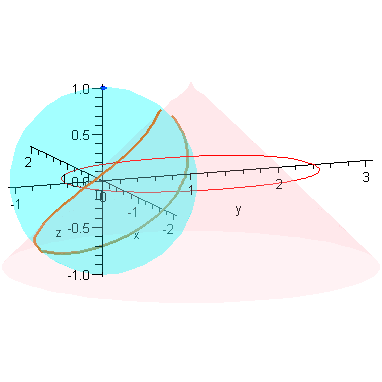>

 >